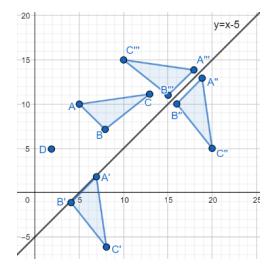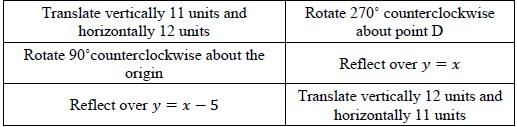# MA.912.GR.2.3Export Print
Identify a sequence of transformations that will map a given figure onto itself or onto another congruent or similar figure.

### Clarifications

Clarification 1: Transformations include translations, dilations, rotations and reflections described using words or using coordinates.

Clarification 2: Within the Geometry course, figures are limited to triangles and quadrilaterals and rotations are limited to 90°, 180° and 270° counterclockwise or clockwise about the center of rotation.

Clarification 3: Instruction includes the understanding that when a figure is mapped onto itself using a reflection, it occurs over a line of symmetry.

General Information
Subject Area: Mathematics (B.E.S.T.)
Strand: Geometric Reasoning
Status: State Board Approved

## Benchmark Instructional Guide

### Terms from the K-12 Glossary

• Coordinate Plane
• Dilation
• Origin
• Reflection
• Rigid Transformation
• Rotation
• Scale Factor
• Translation

### Vertical Alignment

Previous Benchmarks

Next Benchmarks

### Purpose and Instructional Strategies

In the elementary grades, students learned about lines of symmetry. In grade 8, students learned about the effects of translations, rotations, reflections, and dilations on geometric figures. In Geometry, students use their knowledge of translations, dilations, rotations and reflections to identify a sequence or composition of transformations that map a triangle or a quadrilateral onto another congruent or similar figure or onto itself, and they connect reflections to lines of symmetry. In later courses, lines of symmetry are identified as key features in graphs of polynomials and trigonometric functions.
• To describe the sequence of transformations, students will need to know how to describe each one of the transformations in the composition using words or using coordinates. In each case, they will specify vertical and horizontal shifts, center and angle of the rotation, clockwise or counterclockwise, line of reflection, center of the dilation and scale factor, when needed. (MTR.3.1)
• Provide multiple opportunities for students to explore mapping a variety of triangles and quadrilaterals onto congruent or similar figures (given the preimage and the image) using both physical exploration (transparencies or patty paper) and virtual exploration when possible. This will allow students to experience multiple compositions of transformations and realize that more than one sequence can be used to map a figure onto another. (MTR.2.1)
• Instruction includes examples where preimages and images partially overlap each other.
• When a sequence includes a dilation, it may be helpful that students identify the dilation first, and then continue to identify any rigid motions that may be needed.
• Students can explore the sequence of a reflection over the $x$-axis followed by a reflection over the $y$-axis (or any sequence of two reflections over axes perpendicular to each other). To help students make the connection between different sequences of transformations, ask “Is there a single transformation that produces the same image as this sequence?” (MTR.5.1)
• To map a figure onto itself, explore the effect of each transformation. Discuss with students the possibilities of using translations or dilations.
• When a reflection maps a figure onto itself, the line of reflection is also a line of symmetry for the figure. Explore the lines of symmetries of isosceles and equilateral triangles, and rectangles, rhombi, squares, isosceles trapezoids and kites.
• When a rotation is used, explore the cases of regular polygons (equilateral triangles and squares) and how to determine the angles of rotation that will map them onto themselves. (MTR.5.1)
• Instruction includes discussing the case of a dilation with a scale factor of 1. Even if this case is considered trivial, it leads the conversation to the relationship between congruence and similarity. If a dilation is a similarity transformation, then it produces an image that is similar to the preimage. But if a dilation with a scale factor of 1 produces an image that is congruent to the preimage, then congruence is a case of similarity. In other words, when two figures are congruent, then they are necessarily similar to each other.
• An extension of this benchmark may be to explore the angle of rotation needed to map a regular polygon of 5 or more sides onto itself.

### Common Misconceptions or Errors

• Students may believe there is only one sequence that will lead to the image. Instead, students should explore the fact that multiple sequences will result in the same image.• Part A. From the list provided, choose and order transformations that could be used to map ΔABC onto ΔA"'B"'C"'.• Part B. Describe the transformation that maps ΔA"B"C" onto ΔA"'B"'C'".

### Instructional Items

Instructional Item 1• Part A. What is the center of the rotation?
• Part B. If the rotation is counterclockwise, how many degrees is the rotation?
• Part C. Describe another transformation that maps quadrilateral ABCD onto quadrilateral A'B'C'D'.

*The strategies, tasks and items included in the B1G-M are examples and should not be considered comprehensive.

## Related Courses

This benchmark is part of these courses.
1200400: Foundational Skills in Mathematics 9-12 (Specifically in versions: 2014 - 2015, 2015 - 2022, 2022 and beyond (current))
1206310: Geometry (Specifically in versions: 2014 - 2015, 2015 - 2022, 2022 and beyond (current))
1206320: Geometry Honors (Specifically in versions: 2014 - 2015, 2015 - 2022, 2022 and beyond (current))
1206315: Geometry for Credit Recovery (Specifically in versions: 2014 - 2015, 2015 - 2022, 2022 and beyond (current))
7912065: Access Geometry (Specifically in versions: 2015 - 2022, 2022 and beyond (current))

## Related Access Points

Alternate version of this benchmark for students with significant cognitive disabilities.
MA.912.GR.2.AP.3: Identify a given sequence of transformations, that includes translations or reflections, that will map a given figure onto itself or onto another congruent figure.

## Related Resources

Vetted resources educators can use to teach the concepts and skills in this benchmark.

## Formative Assessments

Justifying HL Congruence:

Students are asked to use rigid motion to explain why the HL pattern of congruence ensures right triangle congruence.

Type: Formative Assessment

Two Triangles:

Students are asked to describe the transformations that take one triangle onto another.

Type: Formative Assessment

Indicate the Transformations:

Students are asked to describe the transformations that take one triangle onto another.

Type: Formative Assessment

Similarity - 2:

Students are asked to describe a sequence of transformations to show that two polygons are similar.

Type: Formative Assessment

Similarity - 1:

Students are asked to describe a sequence of transformations to show that two polygons are similar.

Type: Formative Assessment

Similarity - 3:

Students are asked to describe a sequence of transformations that demonstrates two polygons are similar.

Type: Formative Assessment

Multistep Congruence:

Students are asked to describe a sequence of rigid motions to demonstrate the congruence of two polygons.

Type: Formative Assessment

Rigid Motion - 3:

Students are asked to describe a rigid motion to demonstrate two polygons are congruent.

Type: Formative Assessment

Rigid Motion - 2:

Students are asked to describe a rigid motion to demonstrate two polygons are congruent.

Type: Formative Assessment

Rigid Motion - 1:

Students are asked to describe a rigid motion to demonstrate that two polygons are congruent.

Type: Formative Assessment

## Original Student Tutorial

Home Transformations:

Learn to describe a sequence of transformations that will produce similar figures. This interactive tutorial will allow you to practice with rotations, translations, reflections, and dilations.

Type: Original Student Tutorial

## Perspectives Video: Professional/Enthusiast

All Circles Are Similar- Especially Circular Pizza!:

What better way to demonstrate that all circles are similar then to use pizzas! Gaines Street Pies explains how all pizza pies are similar through transformations.

Type: Perspectives Video: Professional/Enthusiast

## MFAS Formative Assessments

Indicate the Transformations:

Students are asked to describe the transformations that take one triangle onto another.

Justifying HL Congruence:

Students are asked to use rigid motion to explain why the HL pattern of congruence ensures right triangle congruence.

Multistep Congruence:

Students are asked to describe a sequence of rigid motions to demonstrate the congruence of two polygons.

Rigid Motion - 1:

Students are asked to describe a rigid motion to demonstrate that two polygons are congruent.

Rigid Motion - 2:

Students are asked to describe a rigid motion to demonstrate two polygons are congruent.

Rigid Motion - 3:

Students are asked to describe a rigid motion to demonstrate two polygons are congruent.

Similarity - 1:

Students are asked to describe a sequence of transformations to show that two polygons are similar.

Similarity - 2:

Students are asked to describe a sequence of transformations to show that two polygons are similar.

Similarity - 3:

Students are asked to describe a sequence of transformations that demonstrates two polygons are similar.

Two Triangles:

Students are asked to describe the transformations that take one triangle onto another.

## Original Student Tutorials Mathematics - Grades 9-12

Home Transformations:

Learn to describe a sequence of transformations that will produce similar figures. This interactive tutorial will allow you to practice with rotations, translations, reflections, and dilations.

## Student Resources

Vetted resources students can use to learn the concepts and skills in this benchmark.

## Original Student Tutorial

Home Transformations:

Learn to describe a sequence of transformations that will produce similar figures. This interactive tutorial will allow you to practice with rotations, translations, reflections, and dilations.

Type: Original Student Tutorial

## Parent Resources

Vetted resources caregivers can use to help students learn the concepts and skills in this benchmark.